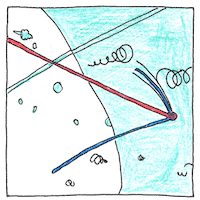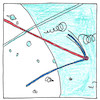# 1956 Neutrino

## The book of science

Tom Sharp

 Wolfgang Pauli, Frederick Reines, Clyde Cowan physics## Neutrino

• In the thirties
• Wolfgang Pauli kept insisting
• there could be a particle
• without an electric charge.
• Pauli called it a neutron
• but Fermi renamed it
• after Chadwick discovered
• neutrons in the nucleus of an atom.
• Assuming that energy
• is conserved, neutrinos
• were needed to explain
• energy lost during beta decay.
• So Reines and Cowan
• set up detectors
• near a nuclear reactor
• and found characteristic
• triggered by neutrinos
• hitting protons
• in water molecules.

## Oscillating mass

• Neutrinos either travel at the speed of light
• or they have a mass, however small.
• If they have mass, they could acquire it
• according to the Majorana mechanism,
• which would mean they are their own antiparticles.
• Multiple experiments have shown
• neutrinos traveling near the speed of light.
• However, they are known to have mass
• because we can observe them oscillate between flavors—
• electron neutrino, muon neutrino, tau neutrino.

## Elusive goal

• Six quarks
• and six leptons
• suggests
• there might be
• exactly three
• neutrino flavors
• although a fourth
• the sterile neutrino
• could exist.

## Theory of light

• Louis de Broglie suggested
• a photon might be a combination
• of a neutrino and an antineutrino
• but the standard theory
• holds that the photon
• is not a composite particle.
• Different problems
• exist with each
• possibility
• but the theory
• of neutrino-antineutrino
• transitions
• underlies the theory
• of flavor oscillation,
• which has been observed.

Sixty-five billion neutrinos from the sun pass through every square centimeter on Earth every second. Boing! They could be hitting many of your protons! Although neutrinos have no electric charge, they interact with other particles by the weak nuclear force. Neutrinos are fermions, along with protons, neutrons, electrons, and quarks.

See also in The book of science: